# Calculus

## Introduction

(I've written this topic specifically for students taking MEI FP2.)

In FP2 you'll need to know how to use the inverse trigonometric functions for differentiation and integration. I'll start by showing you the functions, what their graphs look like, and how to use them in calculus.

Functions need to be one-to-one for you to come up with inverses of them. But obviously the trig functions are periodic. So we make some adjustments to allow them to be invertible.

The inverse sine function $\arcsin x$ is defined for $|x| \le 1$ because $\sin x$ lies between -1 and 1 $$\sin^{-1} x = \arcsin x$$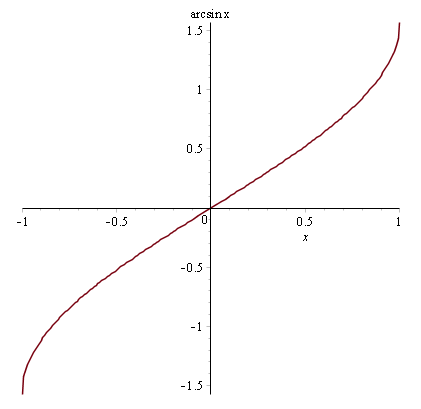The inverse cosine function $\arccos x$ is defined for $|x| \le 1$ because $\cos x$ lies between -1 and 1 $$\cos^{-1} x = \arccos x$$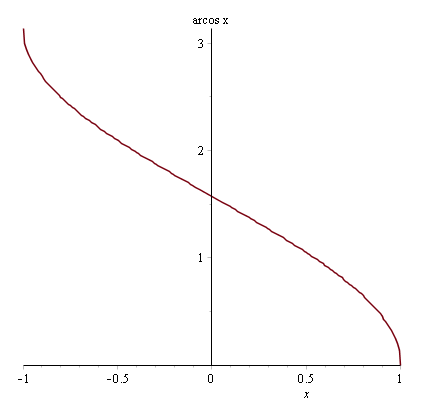The inverse tangent function $\arctan x$ is defined for all $x$ because $\tan x$ goes from $-\infty$ to $\infty$ $$\tan^{-1} x = \arctan x$$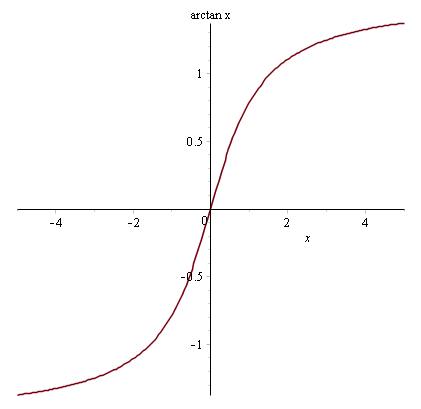### Example

Q) Find $\sin\left(\arctan \left(\frac{3}{4}\right)\right)$.

A) Let $\theta = \arctan (3/4)$, then $\tan \theta = 3/4$. Draw a triangle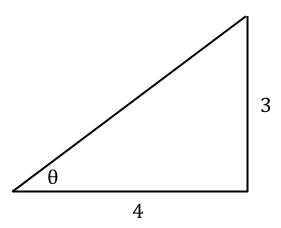Therefore $\sin \theta = \frac{3}{5}$ by the 3-4-5 triangle.

### Example

Q) Find $\cos\left(\arcsin \left(-\frac{5}{6}\right)\right)$.

A) Firstly see that $\arcsin$ is an odd function and $\cos$ is an even function, therefore $$\cos\left(\arcsin \left(-\frac{5}{6}\right)\right) = \cos\left(-\arcsin \left(\frac{5}{6}\right)\right) = \cos\left(\arcsin \left(\frac{5}{6}\right)\right)$$ Let $\theta = \arcsin (5/6)$, then $\sin \theta = 5/6$. Draw a triangle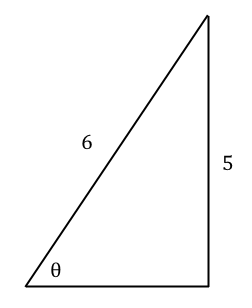The unknown adjacent length is $\sqrt{6^2-5^2} = \surd{11}$. Therefore $\cos \theta = \surd{11}/6$.

## Standard integrals

You need to be familiar with integrals of the following form $$\int \frac{1}{a^2 + x^2} \mathop{dx} = \frac{1}{a} \arctan \left(\frac{x}{a}\right) + c$$ $$\int \frac{1}{\sqrt{a^2 - x^2}} \mathop{dx} = \arcsin \left(\frac{x}{a}\right) + c$$ where $c$ is a constant of integration.

However on the exam it won't always be in this standard form. You'll need to do some quick algebra to get it into the above form.

### Example

Q) Evaluate $\int \frac{1}{25 + 4x^2} \mathop{dx}$.

A) Factorise out 4 in the denominator to get it into the standard form $$\int \frac{1}{25 + 4x^2} \mathop{dx} = \frac{1}{4}\int \frac{1}{\left(\frac{25}{4} + x^2\right)} \mathop{dx} = \frac{1}{10}\arctan\left(\frac{2}{5}x\right) + c$$ where $c$ is a constant of integration.

### Example

Q) Evaluate $\int \frac{1}{\sqrt{8-2x-x^2}} \mathop{dx}$.

A) Complete the square in the denominator $$8 - 2x - x^2 = 8-\left(2x+x^2\right) = 8-\left(\left(x+1\right)^2-1\right) = 9-\left(x+1\right)^2$$ Then the integral is in the standard form. Let $u=x+1$ so that $\frac{du}{dx} = 1$ $$\int \frac{1}{\sqrt{9-(x+1)^2}} \mathop{dx} = \int \frac{1}{\sqrt{9-u^2}} \mathop{du} = \arcsin \left(\frac{u}{3}\right) + c = \arcsin \left(\frac{x+1}{3}\right) + c$$ where $c$ is a constant of integration.

### Example

Q) Evaluate $\int_{0}^{2} \frac{1}{\left(2+\frac{1}{2}x^2\right)} \mathop{dx}$.

A) Factorise out $1/2$ in the denominator to get it into standard form $$\int_{0}^{2} \frac{1}{\left(2+\frac{1}{2}x^2\right)} \mathop{dx} = \int_{0}^{2} \frac{2}{4+x^2} \mathop{dx} = \left[\frac{2}{2}\arctan\left(\frac{x}{2}\right) \right]_{0}^{2} = \arctan(1) - 0 = \frac{\pi}{4}$$

### Example

Q) Evaluate $\int_{2}^{4} \frac{1}{\sqrt{\left(4+x\right)\left(4-x\right)}} \mathop{dx}$.

A) Notice that the denominator has the difference of two squares $$\left(4+x\right)\left(4-x\right) = 16 - x^2$$ So we can put the integral in standard form $$\int_{2}^{4} \frac{1}{\sqrt{16 - x^2}} \mathop{dx} = \left[\arcsin \left(\frac{x}{4}\right)\right]_{2}^{4} = \arcsin(1)-\arcsin\left(\frac{1}{2}\right) = \frac{\pi}{2}-\frac{\pi}{6} = \frac{\pi}{3}$$